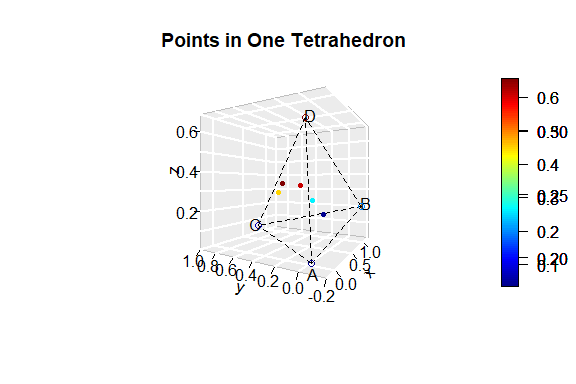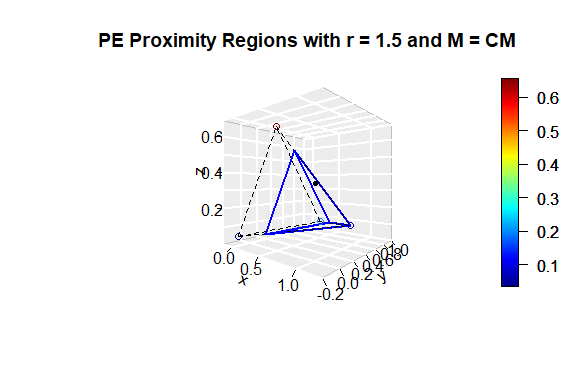# VS2.3 - Illustration of PCDs in One Tetrahedron

#### 2023-06-13

First we load the pcds package:

library(pcds)

# 1 Functions for PCDs with Vertices in One Tetrahedron

For illustration of construction of PCDs and related quantities, we first focus on one Delaunay cell, which is a tetrahedron in the 3D setting. Due to geometry invariance of PE- and CS-PCDs with vertices from uniform distribution in a tetrahedron in $$\mathbb R^3$$, most data generation and computations can be done in the standard regular tetrahedron, and for AS-PCD, one can restrict attention to the basic tetrahedron. Here, we only consider the PE-PCD with vertices being 3D data points, extension of the other PCDs is left for future work. The standard regular tetrahedron is $$T_{reg}=T(A,B,C,D)$$ with vertices $$A=(0,0,0)$$, $$B=(1,0,0)$$, and $$C=\left(1/2,\sqrt{3}/2,0\right)$$, and $$D=\left(1/2,\sqrt{3}/6,\sqrt{6}/3\right)$$.

For more detail on the extension of PCDs to higher dimensions, see Ceyhan (2005), Ceyhan, Priebe, and Wierman (2006), Ceyhan, Priebe, and Marchette (2007), and Ceyhan and Priebe (2007).

We first choose an arbitrary tetrahedron. We choose the arbitrary tetrahedron $$T=T(A,B,C,D)$$ with vertices jittered around the vertices of the standard regular tetrahedron (for better visualization).

set.seed(1)
A<-c(0,0,0)+runif(3,-.25,.25);
B<-c(1,0,0)+runif(3,-.25,.25);
C<-c(1/2,sqrt(3)/2,0)+runif(3,-.25,.25);
D<-c(1/2,sqrt(3)/6,sqrt(6)/3)+runif(3,-.25,.25)
tetra<-rbind(A,B,C,D)
n<-5  #try also n<-10 or 20

And we generate $$n=$$ 5 $$\mathcal{X}$$ points uniformly in the tetrahedron $$T$$ using the function runif.tetra in pcds. One can use the center of mass (default) or the circumcenter of the tetrahedron to construct the vertex regions. $$\mathcal{X}$$ points are denoted as Xp and $$\mathcal{Y}$$ points correspond to the vertices of the tetrahedron $$T$$ (i.e. $$\{A,B,C,D\}$$).

Xp<-runif.tetra(n,tetra)$g #try also Xp[,1]<-Xp[,1]+1 Plot of the tetrahedron $$T$$ and the $$\mathcal{X}$$ points in it, and we also add the vertex names using the text3D function from package plot3D. xlim<-range(tetra[,1],Xp[,1]) ylim<-range(tetra[,2],Xp[,2]) zlim<-range(tetra[,3],Xp[,3]) xr<-xlim-xlim yr<-ylim-ylim zr<-zlim-zlim plot3D::scatter3D(Xp[,1],Xp[,2],Xp[,3], phi=0,theta=-60, bty = "g",main="Points in One Tetrahedron", xlab="x", ylab="y", zlab="z", xlim=xlim+xr*c(-.05,.05), ylim=ylim+yr*c(-.05,.05), zlim=zlim+zr*c(-.05,.05), pch = 20, cex = 1, ticktype = "detailed") #add the vertices of the tetrahedron plot3D::points3D(tetra[,1],tetra[,2],tetra[,3], add = TRUE) A<-tetra[1,]; B<-tetra[2,]; C<-tetra[3,]; D<-tetra[4,] L<-rbind(A,A,A,B,B,C); R<-rbind(B,C,D,C,D,D) plot3D::segments3D(L[,1], L[,2], L[,3], R[,1], R[,2],R[,3], add=TRUE,lwd=1,lty=2) #now we add the vertex names and annotation txt<-tetra xc<-txt[,1]+c(-.02,.02,-.15,.02) yc<-txt[,2]+c(.02,.02,.02,.02) zc<-txt[,3]+c(-.04,.02,.02,.02) txt.str<-c("A","B","C","D") plot3D::text3D(xc,yc,zc,txt.str, add = TRUE)Figure 1.1: Scatterplot of the uniform $$X$$ points in the tetrahedron $$T$$. ## 1.1 Functions for Proportional Edge PCDs with Vertices in One Tetrahedron We use the same tetrahedron $$T$$ and the generated data above, and choose the expansion parameter $$r=1.5$$ and center M="CM" to illustrate the PE proximity region construction. The function NPEtetra is used for the construction of PE proximity regions taking the arguments p,th,r,M,rv where • p, a 3D point whose PE proximity region is to be computed, • r, a positive real number which serves as the expansion parameter in PE proximity region; must be $$\ge 1$$. • th, A $$4 \times 3$$ matrix with each row representing a vertex of the tetrahedron, • M, the center to be used in the construction of the vertex regions in the tetrahedron, th, Currently it only takes "CC" for circumcenter and "CM" for center of mass; default="CM", • rv, the index of the vertex region containing the point, either 1,2,3,4 (default is NULL). NPEtetra returns the proximity region as the the vertices of the tetrahedron proximity region. M<-"CM" #try also M<-"CC" r<-1.5 NPEtetra(Xp[1,],tetra,r) #uses the default M="CM" #> [,1] [,2] [,3] #> [1,] 0.72233763 0.9464243 0.06455702 #> [2,] 0.06169821 0.1514047 0.04242222 #> [3,] 1.10142305 0.0843472 0.17049892 #> [4,] 0.37498004 0.3131847 0.52897936 Indicator for the presence of an arc from a (data or $$\mathcal{X}$$) point to another for PE-PCDs is the function IarcPEtetra which takes arguments p1,p2,th,r,M,rv where • p1, a 3D point whose PE proximity region is constructed. • p2, a 3D point. The function determines whether p2 is inside the PE proximity region of p1 or not. • th,r,M,rv are as in NPEtetra. This function returns $$I(p_2 \in N_{PE}(p_1,r))$$, that is, returns 1 if p2 is in $$N_{PE}(p_1,r)$$, 0 otherwise. One can use it for points in the data set or for arbitrary points (as if they were in the data set). IarcPEtetra(Xp[1,],Xp[2,],tetra,r) #uses the default M="CM" #>  1 IarcPEtetra(Xp[2,],Xp[1,],tetra,r,M) #>  1 Number of arcs of the PE-PCD can be computed by the function num.arcsPEtetra, which is an object of class “NumArcs” and takes arguments Xp,th,r,M where Xp is the data set, and th,r,M are as in NPEtetra. This function returns the list of • desc: A description of the PCD and the output • num.arcs: Number of arcs of the PE-PCD, • num.in.tetra: Number of Xp points in the tetrahedron tetra, • ind.in.tetra: The vector of indices of the Xp points that reside in the tetrahedron. • tess.points: Vertices of the tetrahedron (i.e. Yp points) • vertices: vertices of the PCD (i.e. Xp points) The output is as in num.arcsPEtri. Narcs = num.arcsPEtetra(Xp,tetra,r,M) summary(Narcs) #> Call: #> num.arcsPEtetra(Xp = Xp, th = tetra, r = r, M = M) #> #> Description of the output: #> Number of Arcs of the PE-PCD with vertices Xp and Quantities Related to the Support Tetrahedron #> #> Number of data (Xp) points in the tetrahedron = 5 #> Number of arcs in the digraph = 10 #> #> Indices of data points in the tetrahedron: #> 1 2 3 4 5 #> #plot(Narcs) #gives error The arc density of the PE-PCD can be computed by the function PEarc.dens.tetra. It takes the arguments Xp,th,r,M,th.cor where Xp,th,r,M are as in num.arcsPEtetra and th.cor is a logical argument for computing the arc density for only the points inside the tetrahedron, th. (default is th.cor=FALSE), i.e., if th.cor=TRUE only the induced digraph with the vertices inside th are considered in the computation of arc density. Arc density can be adjusted (or corrected) for the points outside the triangle by using the option th.cor=TRUE. PEarc.dens.tetra(Xp,tetra,r,M) #>  0.5 The incidence matrix of the PE-PCD for the one tetrahedron case can be found by inci.matPEtetra, using the inci.matPEtetra(Xp,tetra,r,M) command. It has the same arguments as num.arcsPEtetra function. Plots of the PE proximity region for one of the $$\mathcal{X}$$ points only (for better visualization). plotPEregs.tetra(Xp[1,],tetra,r=1.5) #uses the default M="CM"Figure 1.2: PE proximity regions for one of the $$X$$ points in the tetrahedron $$T$$ for better visualization. ## 1.2 Functions for Construction and Simulation of Proportional Edge PCDs in the Standard Regular Tetrahedron We first define the standard regular tetrahedron $$T_{reg}$$ as in Section 1. A<-c(0,0,0); B<-c(1,0,0); C<-c(1/2,sqrt(3)/2,0); D<-c(1/2,sqrt(3)/6,sqrt(6)/3) tetra<-rbind(A,B,C,D) n<-10 #try also n<-20 And we generate $$\mathcal{X}$$ points of size $$n=$$ 5 using the function runif.std.tetra in the pcds package, and use either center of mass (default) or the circumcenter of $$T_{reg}$$ to construct vertex regions. Xp<-runif.std.tetra(n)$g

Plot of the standard regular tetrahedron $$T_{reg}$$ and the $$\mathcal{X}$$ points in it are provided using the below code. We also add the vertex names using the text3D function from package plot3D.

xlim<-range(tetra[,1],Xp[,1])
ylim<-range(tetra[,2],Xp[,2])
zlim<-range(tetra[,3],Xp[,3])

xr<-xlim-xlim
yr<-ylim-ylim
zr<-zlim-zlim

plot3D::scatter3D(Xp[,1],Xp[,2],Xp[,3], phi=0,theta=-60, bty = "g",main="Points in the Standard Regular Tetrahedron",
xlab="x", ylab="y", zlab="z", xlim=xlim+xr*c(-.05,.05), ylim=ylim+yr*c(-.05,.05),
zlim=zlim+zr*c(-.05,.05), pch = 20, cex = 1, ticktype = "detailed")
#add the vertices of the tetrahedron
A<-tetra[1,]; B<-tetra[2,]; C<-tetra[3,]; D<-tetra[4,]
L<-rbind(A,A,A,B,B,C); R<-rbind(B,C,D,C,D,D)
plot3D::segments3D(L[,1], L[,2], L[,3], R[,1], R[,2],R[,3], add=TRUE,lwd=1,lty=2)

#now we add the vertex names and annotation
txt<-tetra
xc<-txt[,1]+c(-.01,.02,-.12,.02)
yc<-txt[,2]+c(.02,.02,.02,-.01)
zc<-txt[,3]+c(-.04,.02,.02,.02)
txt.str<-c("A","B","C","D")
plot3D::text3D(xc,yc,zc,txt.str, add = TRUE)

The function NPEstd.tetra is used for the construction of PE proximity regions for points in $$T_{reg}$$ taking arguments p,r,rv which are same as in NPEtetra. This function returns the proximity region as the the vertices of the tetrahedron proximity region. Center of mass is equivalent to circumcenter for $$T_{reg}$$, so no argument is specified for the center.

r<-1.5
NPEstd.tetra(Xp[1,],r)
#>           [,1]      [,2]      [,3]
#> [1,] 0.5000000 0.8660254 0.0000000
#> [2,] 0.1618881 0.2803983 0.0000000
#> [3,] 0.5000000 0.4756074 0.5521345
#> [4,] 0.8381119 0.2803983 0.0000000
NPEstd.tetra(Xp[5,],r)
#>            [,1]      [,2]      [,3]
#> [1,] 1.00000000 0.0000000 0.0000000
#> [2,] 0.52499658 0.8227301 0.0000000
#> [3,] 0.52499658 0.2742434 0.7756773
#> [4,] 0.04999316 0.0000000 0.0000000

Indicator for the presence of an arc from a (data or $$\mathcal{X}$$) point to another for PE-PCDs is the function IarcPEstd.tetra which takes arguments p1,p2,r,rv and returns $$I(p_2 \in N_{PE}(p_1,r))$$ which are same as in IarcPEtetra. One can use it for points in the data set or for arbitrary points (as if they were in the data set).

IarcPEstd.tetra(Xp[1,],Xp[3,],r)  #uses the default M="CM"
#>  1

Plots of the PE proximity regions for all points can be obtained with the below code:

plotPEregs.std.tetra(Xp,r)

## 1.3 Auxiliary Functions to Define Proximity Regions for Points in a Tetrahedron

We only use circumcenter (CC) or center of mass (CM) to construct vertex regions in a tetrahedron, and here we illustrate finding CC (as CM is just the componentwise arithmetic average of the vertices) of a tetrahedron. The function circumcenter.tetra takes the sole argument th for a $$4 \times 3$$ matrix with each row representing a vertex of the tetrahedron and returns the circumcenter of a general tetrahedron. We use a tetrahedron $$T$$ whose vertices are jittered around the vertices of a standard regular tetrahedron for better visualization in the plots.

set.seed(123)
A<-c(0,0,0)+runif(3,-.2,.2);
B<-c(1,0,0)+runif(3,-.2,.2);
C<-c(1/2,sqrt(3)/2,0)+runif(3,-.2,.2);
D<-c(1/2,sqrt(3)/6,sqrt(6)/3)+runif(3,-.2,.2);
tetra<-rbind(A,B,C,D)

CC<-circumcenter.tetra(tetra)
CC
#>  0.5516851 0.3386671 0.1212977

We can also illustrate the CC in a tetrahedron in a plot with vertex and CC annotation. Type ? circumcenter.tetra for the code to generate the corresponding figure.

The function rel.vert.tetraCC takes arguments p,th and returns the index of the $$CC$$-vertex region in a tetrahedron th where the point p resides.

n<-10  #try also n<-20
Xp<-runif.tetra(n,tetra)$g rel.vert.tetraCC(Xp[1,],tetra) #>$rv
#>  2
#>
#> $tetra #> [,1] [,2] [,3] #> vertex 1 -0.08496899 0.1153221 -0.03640923 #> vertex 2 1.15320696 0.1761869 -0.18177740 #> vertex 3 0.51124220 1.0229930 0.02057401 #> vertex 4 0.48264589 0.4714085 0.79783024 Rv<-vector() for (i in 1:n) Rv<-c(Rv,rel.vert.tetraCC(Xp[i,],tetra)$rv)
Rv
#>   2 2 1 3 2 1 2 3 2 1

We also generate $$n=$$ 5 $$\mathcal{X}$$ points uniformly in the tetrahedron and find the indices of the vertex regions they reside in.

We illustrate the CC-vertex regions using the following code. We annotate the vertices and CC and provide the scatterplot of these points labeled according to the vertex region they reside in. Type also ? rel.vert.tetraCC for the code to generate this figure.

CC<-circumcenter.tetra(tetra)
CC

Xlim<-range(tetra[,1],Xp[,1],CC)
Ylim<-range(tetra[,2],Xp[,2],CC)
Zlim<-range(tetra[,3],Xp[,3],CC)
xd<-Xlim-Xlim
yd<-Ylim-Ylim
zd<-Zlim-Zlim

plot3D::scatter3D(tetra[,1],tetra[,2],tetra[,3], phi =0,theta=40, bty = "g",
main="Scatterplot of data points with CC-vertex regions",
xlim=Xlim+xd*c(-.05,.05),ylim=Ylim+yd*c(-.05,.05), zlim=Zlim+zd*c(-.05,.05),
pch = 20, cex = 1, ticktype = "detailed")
L<-rbind(A,A,A,B,B,C); R<-rbind(B,C,D,C,D,D)
plot3D::segments3D(L[,1], L[,2], L[,3], R[,1], R[,2],R[,3], add=TRUE,lwd=2)

D1<-(A+B)/2; D2<-(A+C)/2; D3<-(A+D)/2; D4<-(B+C)/2; D5<-(B+D)/2; D6<-(C+D)/2;
L<-rbind(D1,D2,D3,D4,D5,D6); R<-matrix(rep(CC,6),ncol=3,byrow=TRUE)
plot3D::segments3D(L[,1], L[,2], L[,3], R[,1], R[,2],R[,3], add=TRUE,lty=2)

F1<-intersect.line.plane(A,CC,B,C,D)
L<-matrix(rep(F1,4),ncol=3,byrow=TRUE); R<-rbind(D4,D5,D6,CC)
plot3D::segments3D(L[,1], L[,2], L[,3], R[,1], R[,2],R[,3],col=2, add=TRUE,lty=2)

F2<-intersect.line.plane(B,CC,A,C,D)
L<-matrix(rep(F2,4),ncol=3,byrow=TRUE); R<-rbind(D2,D3,D6,CC)
plot3D::segments3D(L[,1], L[,2], L[,3], R[,1], R[,2],R[,3],col=3, add=TRUE,lty=2)

F3<-intersect.line.plane(C,CC,A,B,D)
L<-matrix(rep(F3,4),ncol=3,byrow=TRUE); R<-rbind(D3,D5,D6,CC)
plot3D::segments3D(L[,1], L[,2], L[,3], R[,1], R[,2],R[,3],col=4, add=TRUE,lty=2)

F4<-intersect.line.plane(D,CC,A,B,C)
L<-matrix(rep(F4,4),ncol=3,byrow=TRUE); R<-rbind(D1,D2,D4,CC)
plot3D::segments3D(L[,1], L[,2], L[,3], R[,1], R[,2],R[,3],col=5, add=TRUE,lty=2)

plot3D::text3D(Xp[,1],Xp[,2],Xp[,3], labels=factor(Rv), add=TRUE)

The function rel.vert.tetraCM takes the same arguments as rel.vert.tetraCC and returns the index of the $$CM$$-vertex region in a tetrahedron th where the point p resides. We use standard regular tetrahedron in this example.

#The index of the $CM$-vertex region in a tetrahedron that contains a point
A<-c(0,0,0); B<-c(1,0,0); C<-c(1/2,sqrt(3)/2,0); D<-c(1/2,sqrt(3)/6,sqrt(6)/3)
tetra<-rbind(A,B,C,D)

n<-10  #try also n<-20
Xp<-runif.std.tetra(n)$g rel.vert.tetraCM(Xp[1,],tetra) #>$rv
#>  4
#>
#> $tetra #> [,1] [,2] [,3] #> vertex 1 0.0 0.0000000 0.0000000 #> vertex 2 1.0 0.0000000 0.0000000 #> vertex 3 0.5 0.8660254 0.0000000 #> vertex 4 0.5 0.2886751 0.8164966 Rv<-vector() for (i in 1:n) Rv<-c(Rv, rel.vert.tetraCM(Xp[i,],tetra)$rv )
Rv
#>   4 4 3 4 4 1 1 3 3 2

We also generate $$n=$$ 5 $$\mathcal{X}$$ points in the tetrahedron and find the indices of the vertex regions they reside in.

We can also illustrate the CC in a tetrahedron in a plot with vertex and CC annotation. Type ? rel.vert.tetraCM for the code to generate the corresponding figure.